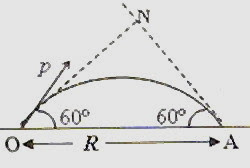## Saturday, February 28, 2009

### Angular Momentum- Two Multiple Choice Questions

How strange is the lot of us mortals! Each of us is here for a brief sojourn; for what purpose we know not, though some times sense it. But we know from daily life that we exist for other people first of all for whose smiles and well-being our happiness depends.

– Albert Einstein

(1) A thin uniform wooden rod AB of length L and mass M is hinged without friction at the end B. A small block of clay of mass m moving horizontally with velocity v srikes the end B of the rod and gets stuck to it. The angular velocity of the system about A just after the collision is

(b) 3mv/(mL + ML)

(c) (mv+ ML)/ 3mL

(d) mv/(3mL + ML)

(e) 3mv/(3mL + ML)

The angular momentum of the system about the point A just before collision is the angular momentum of the block of clay which is equal to mvL. (Note that the perpendicular distance of the line of action of the linear momentum (mv) of the block of clay from the point A is L).

The angular momentum of the system about the point A just after the collision is where I is the total moment of inertia of the rod and clay and ω is the angular velocity of the system immediately after the collision.

We have I = ML2/3 + mL2

Equating the angular momenta before and after collision, we have

mvL = (ML2/3 + mL2) ω

Therefore ω = 3mv/(3mL + ML).

(2) A particle is projected at an angle of 60º with the horizontal with linear momentum of magnitude p. The horizontal range of this projectile is R. Just before the projectile strikes the ground at A, what is the magnitude of its angular momentum aboutan axis perpendicular the plane of motion and passing through the point of projection? Neglect air resistance.

(a) pR

(b) pR/2

(c) (√3) pR /2

(d) (√3) pR

(e) Zero

This is a very simple question. The magnitude of the linear momentum of the projectile just before it strikes the ground will be equal to p. The magnitude of the angular momentum at the moment will be p×Lever arm = p×ON = p×R sin 60º = (√3) pR /2.

## Wednesday, February 11, 2009

### IIT-JEE 2008 Linked Comprehension Type Multiple Choice Questions Inviolving Thermal Physics and Properties of Fluids

Today we will discuss three linked comprehension type multiple choice questions which appeared in IIT-JEE 2008 question paper:

Paragraph for Question Nos. 1 to 3A small spherical monoatomic ideal gas bubble (γ = 5/3) is trapped inside a liquid of density ρ (see figure). Assume that the bubble does not exchange any heat with the liquid. The bubble contains n moles of gas. The temperature of the gas when the bubble is at the bottom is T0, the height of the liquid is H and the atmospheric pressure is P0 (Neglect surface tension).

1. As the bubble moves upwards, besides the buoyancy force the following forces are acting on it:

(A) Only the force of gravity

(B) The force due to gravity and the force due to the pressure of the liquid

(C) The force due to gravity, the force due to the pressure of the liquid and the force due to viscosity of the liquid

(D) The force due to gravity and the force due to viscosity of the liquid

The force due to the pressure of the liquid is the buoyancy force. Therefore, besides the buoyancy force the forces acting on the bubble are the force due to gravity and the force due to viscosity of the liquid [Option (D)].

2. When the gas bubble is at height y from the bottom, its temperature is

(A) T0[(P0 + ρ gH) /(P0 + ρ gy)]2/5

(B) T0[{P0 + ρ g(H y)}/(P0 + ρ gH)]2/5

(C) T0[(P0 + ρ gH) /(P0 + ρ gy)]3/5

(D) T0[{P0 + ρ g(H y)}/(P0 + ρ gH)]3/5

Since there is no heat exchange, the process is adiabatic for which

T P[(1- γ)/ γ] = constant where γ is the ratio of specific heats.

[Many of you might be remembering this as T γ P(1- γ) = constant].

At the bottom pressure = P0 + ρ gH and temperature = T0

At height y from the bottom pressure = P0 + ρ g(H y) and temperature = T (let us say)

Therefore, T0(P0 + ρ gH)–2/5 = T[P0 + ρ g(H y)]–2/5 so that

T = T0[(P0 + ρ gH) /{P0 + ρ g(H y)}]–2/5

Or, T = T0 [{P0 + ρ g(H y)}/(P0 + ρ g(H )]2/5

So option (B) is correct.

3. The buoyancy force on the gas bubble is (Assume R is the universal gas constant)

(A) ρ nRgT0 [(P0 + ρ gH) 2/5/(P0 + ρ gy) 7/5]

(B) ρ nRgT0 /[(P0 + ρ gH) 2/5{P0 + ρ g(H y)}3/5]

(C) ρ nRgT0 [(P0 + ρ gH) 3/5/(P0 + ρ gy) 8/5]

(D) ρ nRgT0 /[(P0 + ρ gH) 3/5{P0 + ρ g(H y)}2/5]

Force of buoyancy, F= g

But V = nRT/P so that F = nRTρg /P

Here P = P0 + ρ g(H y) and T = T0 [{P0 + ρ g(H y)}/(P0 + ρ g(H )]2/5

Substituting, F = nRρg T0 [{P0 + ρ g(H y)}/(P0 + ρ g(H )]2/5/ [P0 + ρ g(H y)]

Or, F = ρ nRgT0 /[(P0 + ρ gH) 2/5{P0 + ρ g(H y)}3/5]

So option (B) is correct.